• 1:积分电路可以使输入方波转换成三角波或者斜波 微分电路可以使输入方波转换成尖脉冲波 2:积分电路电阻串联在主电路中，电容在干路中 微分则相反 3：积分电路的时间常数t要大于或者等于10倍输入脉冲宽度 微分电路的...
1:积分电路可以使输入方波转换成三角波或者斜波
微分电路可以使输入方波转换成尖脉冲波
2:积分电路电阻串联在主电路中，电容在干路中
微分则相反
3：积分电路的时间常数t要大于或者等于10倍输入脉冲宽度
微分电路的时间常数t要小于或者等于1/10倍的输入脉冲宽度
4：积分电路输入和输出成积分关系
微分电路输入和输出成微分关系

展开全文• 实验——一阶RC电路“稳态”与 “暂态”的概念一阶电路过渡过程一阶电路的定义一阶R-C电路的充电过程曲线一阶R-C电路的放电过程曲线时间常数过渡过程曲线微分电路τ值的计算与测量方法积分电路 研究一阶电路在阶跃...
实验——一阶RC电路（试验记录）“稳态”与 “暂态”的概念一阶电路过渡过程一阶电路的定义一阶R-C电路的充电过程曲线一阶R-C电路的放电过程曲线时间常数过渡过程曲线微分电路τ值的计算与测量方法积分电路
研究一阶电路在阶跃信号作用下的过渡过程。
“稳态”与 “暂态”的概念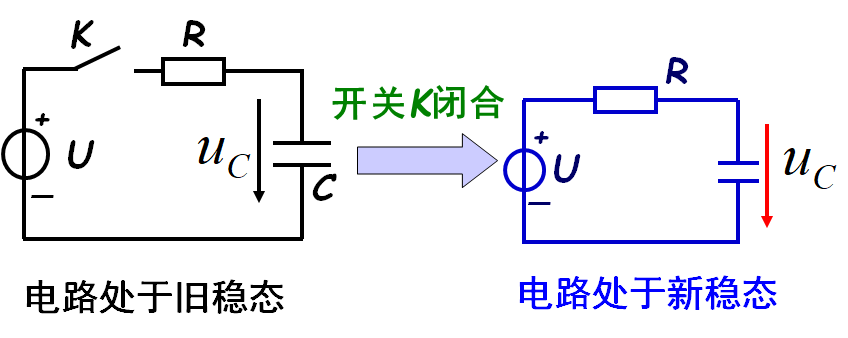暂态过程就是电路由一个稳态向另一个新的稳态转变的过程
一阶电路过渡过程
电阻电路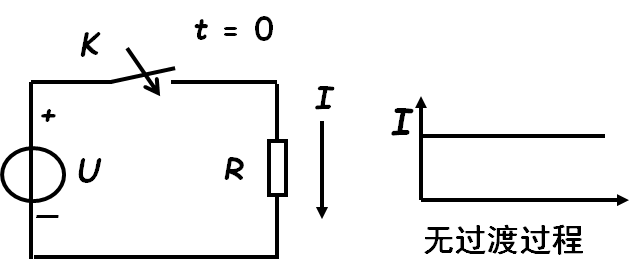电阻是耗能元件，电流随电压比例变化，
不存在过渡过程。
电容电路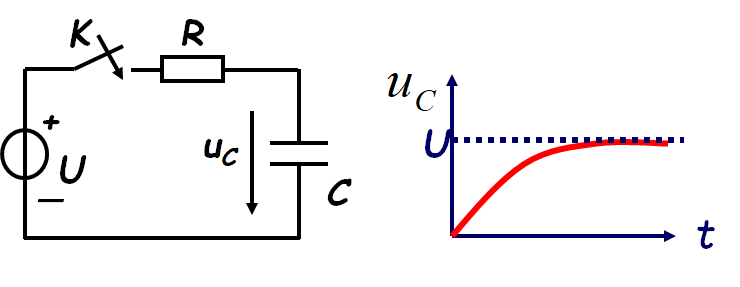电容为储能元件，它储存的能量为电场能量 ，其大小为：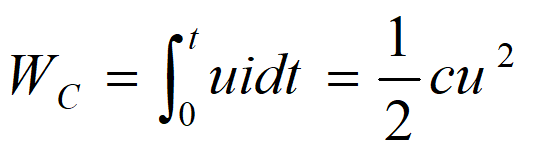因为能量的存储和释放需要一个过程，所以有电容的电路存在过渡过程。
一阶电路的定义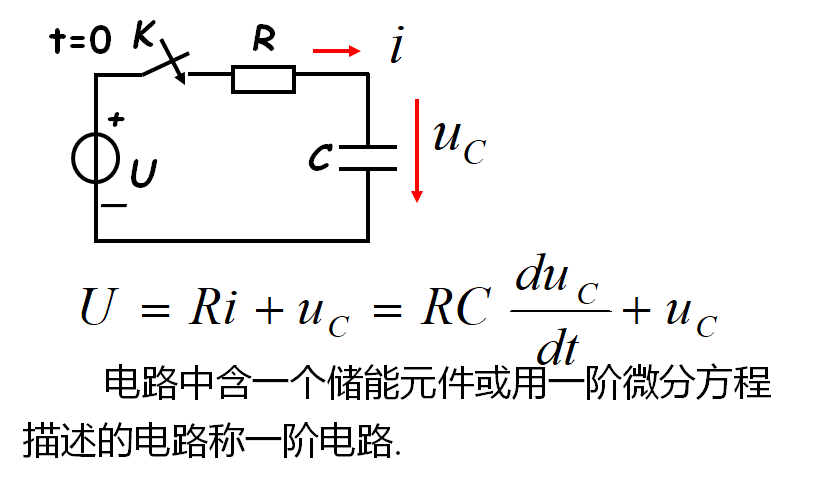一阶R-C电路的充电过程曲线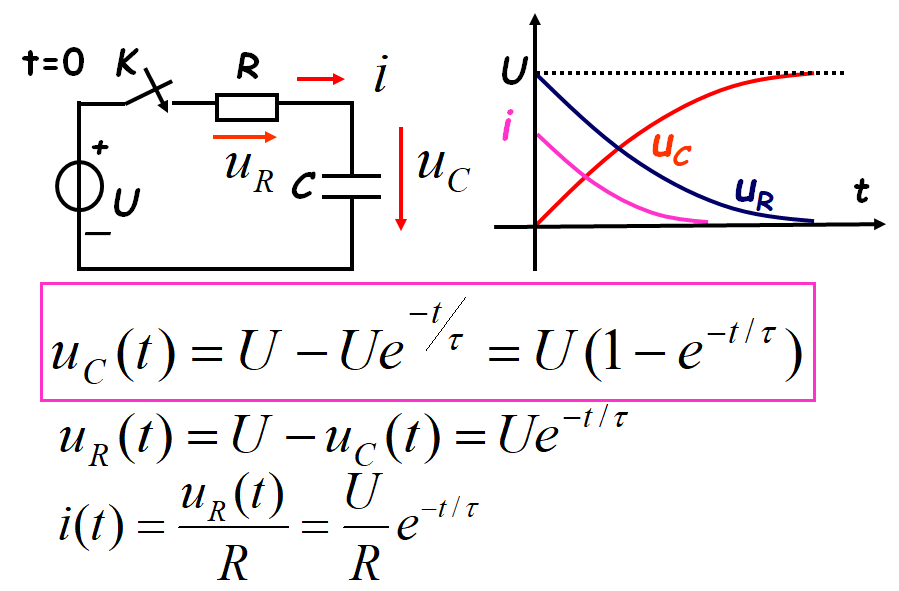一阶R-C电路的放电过程曲线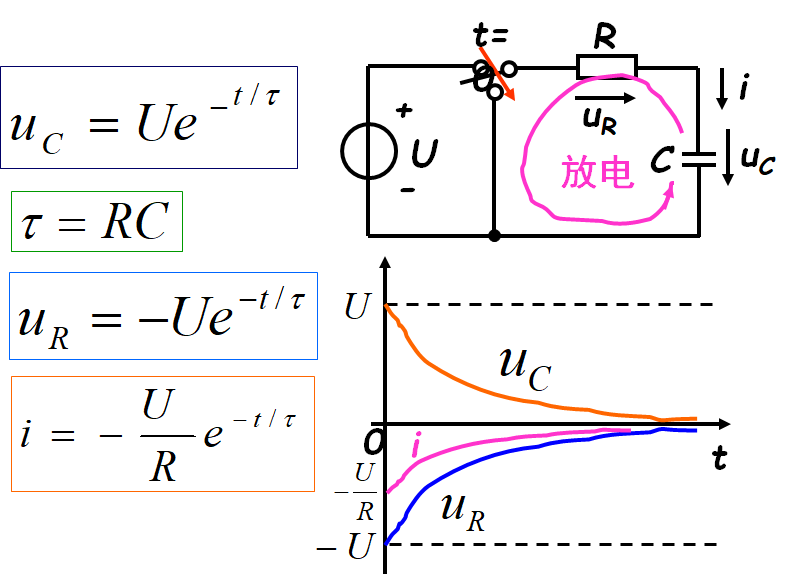时间常数
τ=RC
过渡过程曲线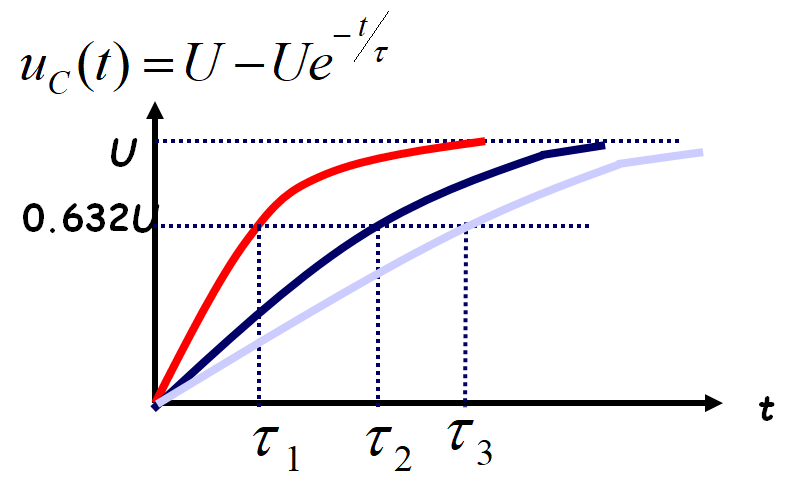**结论：**τ越大，过渡过程曲线变化越慢，uC达到稳态所需要的时间越长。
微分电路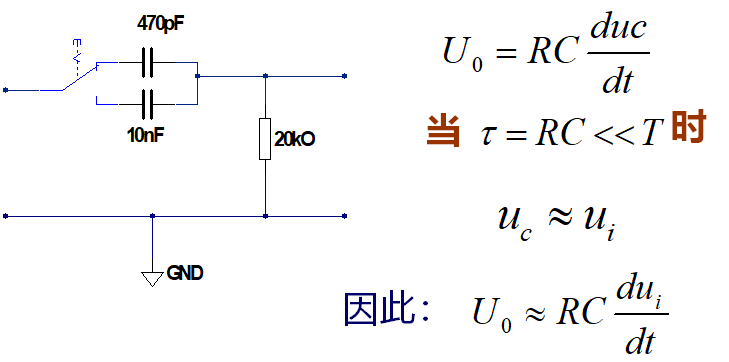即，输出电压的大小近似与输入电压的微分成正比。
所以称该电路为微分电路。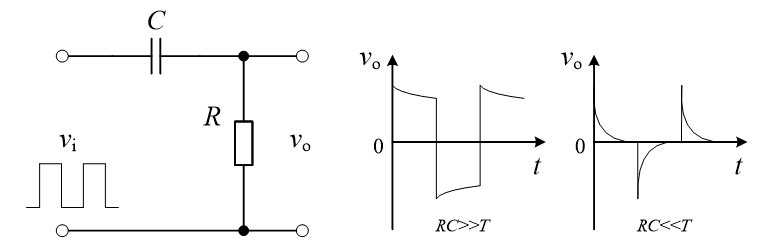τ值的计算与测量方法
τ=RC；
法一：
当τ=t时  U=0.368U0 (V)；从输出曲线上可读出 τ值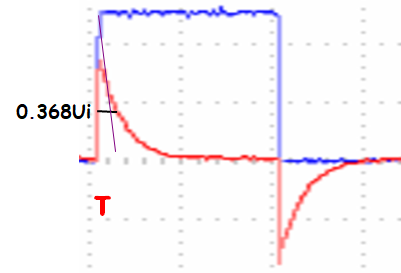法二：
因曲线在t=0点处的切线与横轴的交点也是τ值，所以可通过作一过顶点的直线，求出 τ值
积分电路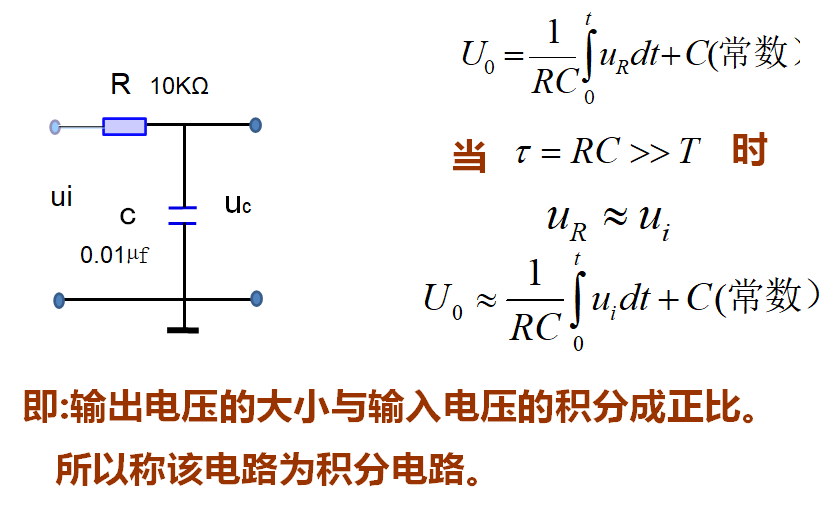展开全文• 输出信号与输入信号的积分成正比的电路：积分电路输出信号与输入信号的微分成正比的电路：微分电路1)一阶RC低通滤波器RC低通滤波器的电路及其幅频、相频特性如下图所示。设滤波器的输入电压为ex输出电压为ey，电路的...


输出信号与输入信号的积分成正比的电路：积分电路输出信号与输入信号的微分成正比的电路：微分电路1)一阶RC低通滤波器RC低通滤波器的电路及其幅频、相频特性如下图所示。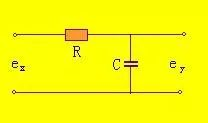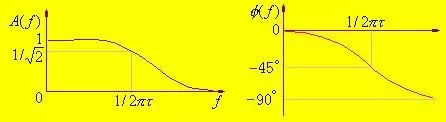设滤波器的输入电压为ex输出电压为ey，电路的微分方程为：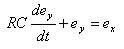这是一个典型的一阶系统。令=RC，称为时间常数，对上式取拉氏变换，有：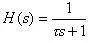或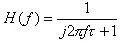其幅频、相频特性公式为：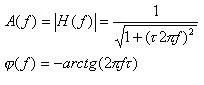分析可知，当f很小时，A(f)=1，信号不受衰减的通过;当f很大时，A(f)=0，信号完全被阻挡，不能通过。2)一阶RC高通滤波器RC高通滤波器的电路及其幅频、相频特性如下图所示。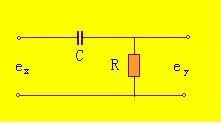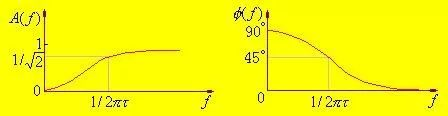设滤波器的输入电压为ex输出电压为ey，电路的微分方程为：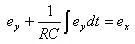同理，令=RC，对上式取拉氏变换，有：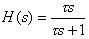或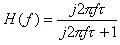其幅频、相频特性公式为：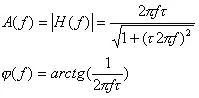分析可知，当f很小时，A(f)=0，信号完全被阻挡，不能通过;当f很大时，A(f)=1信号不受衰减的通过.3)RC带通滤波器带通滤波器可以看作为低通滤波器和高通滤波器的串联，其电路及其幅频、相频特性如下图所示。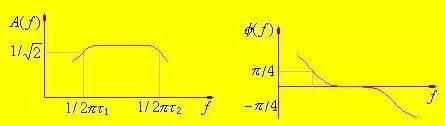其幅频、相频特性公式为： H(s) = H1(s) * H2(s)式中H1(s)为高通滤波器的传递函数，H2(s)为低通滤波器的传递函数。有：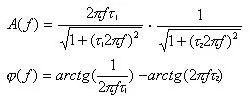这时极低和极高的频率成分都完全被阻挡，不能通过;只有位于频率通带内的信号频率成分能通过。须要注意，当高、低通两级串联时，应消除两级耦合时的相互影响，因为后一级成为前一级的“负载”，而前一级又是后一级的信号源内阻.实际上两级间常用射极输出器或者用运算放大器进行隔离.所以实际的带通滤波器常常是有源的.有源滤波器由RC调谐网络和运算放大器组成.运算放大器既可作为级间隔离作用，又可起信号幅值的放大作用.

展开全文•惯性环节
• 目录动态电路的方程及其初始条件一阶电路零输入响应一阶电路零状态响应一阶电路全响应三要素法二阶电路零输入响应二阶电路零状态响应二阶电路全响应一阶电路和二阶电路的阶跃响应 动态电路的方程及其初始条件 动态...
目录动态电路的方程及其初始条件一阶电路零输入响应一阶电路零状态响应一阶电路全响应三要素法二阶电路过渡过程的判定冲激响应和阶跃响应阶跃响应$ε(t)$冲激响应$δ(t)$
动态电路的方程及其初始条件
动态电路即指含有电容、电感等动态原件的电路，在条件发生变化时（如加入激励源、阶跃响应等），电路响应发生变化。电路响应发生变化的时间很短，在0-—0+之间，这个过渡过程就叫做换路。
所谓动态电路，也即能够用常微分方程来描述的电路，本文讨论分别用一阶常微分方程和二阶常微分方程来描述的一阶动态电路和二阶动态电路。
我们在分析的过程中将 iL(0+) 和 uc(0+) 作为独立的初始条件（在后续的求解中先求出独立的初始条件），其余均为非独立的初始条件。
对于电容：
$u_c(t) = u_c(t_0)+1/C\int_0^t i_cdt$
$u_c(0_+)=u_c(0_-)+1/C\int_{0_-}^{0_+} i_cdt$
对电感：
$i_L(t) = i_L(t_0)+1/L\int_0^tu_Ldt$
$i_L(0_+)=i_L(0_-)+1/L\int_{0_-}^{0_+}u_Ldt$
（上述几个公式很重要，在如冲激激励时可直接套用计算）
由于 ic 有限，uc不能跳变，同理可用于 uL，iL。
于是就有换路定理：
$u_c(0_+)=u_c(0_-)$
$i_L(0_+)=i_L(0_-)$
于是，在换路瞬间，电容可以看作电压源或视为短路；电感可以看作电流源或视为开路。
!!!但在电路达到稳态时，电容视为开路；电感视为短路。
一阶电路零输入响应
所谓零输入响应，就是指在换路后没有激励源激励作用，电路的响应全由动态原件初始储能提供。
具体由RC串联电路和RL并联电路推导解常微分方程可得：
$u_c=u_c(0_+)e^{-\frac{t}{RC}}=U_0e^{-\frac{t}{RC}}$
$i_L=i_L(0_+)e^{-t\frac{R}{L}}=I_0e^{-t\frac{R}{L}}$
此外，电容放电过程中电容电场能全部被电阻吸收转化为热能
$W_R=\frac{1}{2}CU_0^2$
(工程上一般认为 3—5τ 电容放电结束)
一阶电路零状态响应
零状态响应就是动态原件初始储能为0，外加激励源供电路响应。
具体由RC串联电路和RL并联电路推导解常微分方程可得：
$u_c=U_s-U_se^{-\frac{t}{τ}}=U_s(1-e^{-\frac{t}{τ}})$
$i_L=I_s-I_se^{-\frac{t}{τ}}=I_s(1-e^{-\frac{t}{τ}})$
同样有：
$W_R=\frac{1}{2}CU_s^2$
一阶电路全响应
全响应 = 零输入响应 + 零状态响应
ex：
$u_c=U_0e^{-\frac{t}{τ}}+U_s(1-e^{-\frac{t}{τ}})$
或者
全响应 = 强制分量 + 自由分量
全响应 = 稳态分量 + 暂态分量
ex:
$u_c=U_s+(U_0-U_s)e^{-\frac{t}{τ}}$
（考察什么时候电路立即进入稳态，即暂态响应为零）
三要素法
三要素法不同于以上所述经典法，其公式如下：
$f(t)=f(∞)+[f(0_+)-f(∞)]*e^{-t/τ}$
因此只需要求出：

初始值$f(0_+)$
稳态量$f(∞)$
时间常数$τ$（RC、L/R）

即可。
通常，初始值由换路定理确定，稳态量在稳态后（电容开路、电感短路）对电路进行分析求得，时间常数主要在于求R（Req）
注意：三要素法仅适用于一阶电路！
PLUS：
以下情况若左边为电流源则可以等效为两个一阶电路串联，互不影响，分别求解；若为电压源则不能作此等效，具体证明过程就不给出。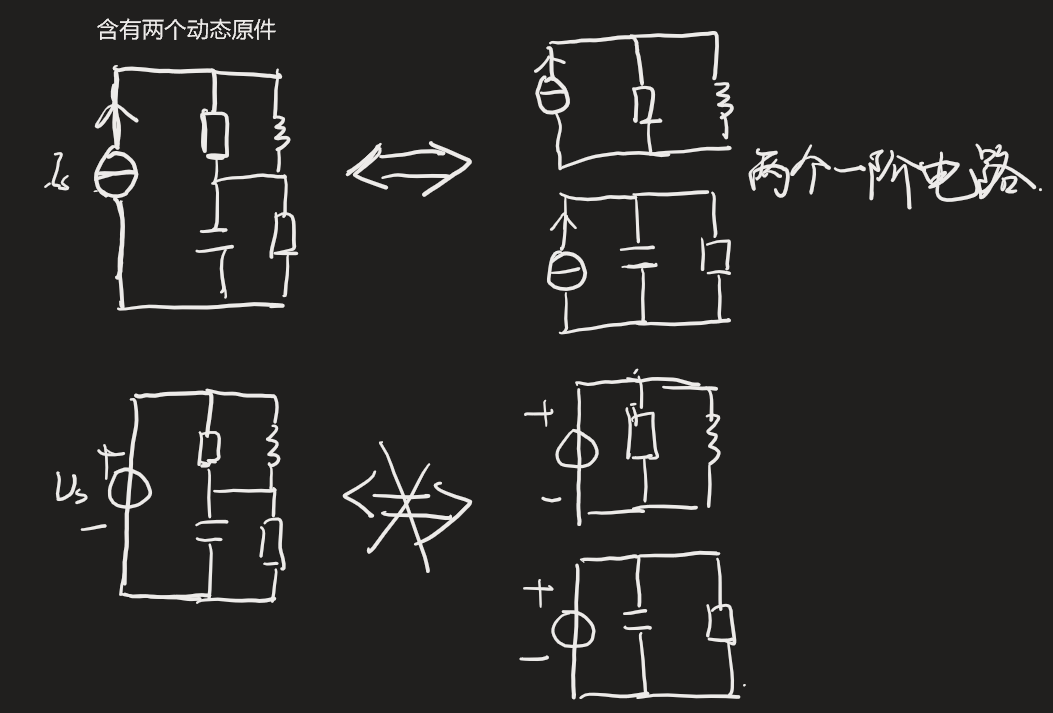同理下图若左边为电压源则可以等效为两个一阶电路并联，互不影响，分别求解。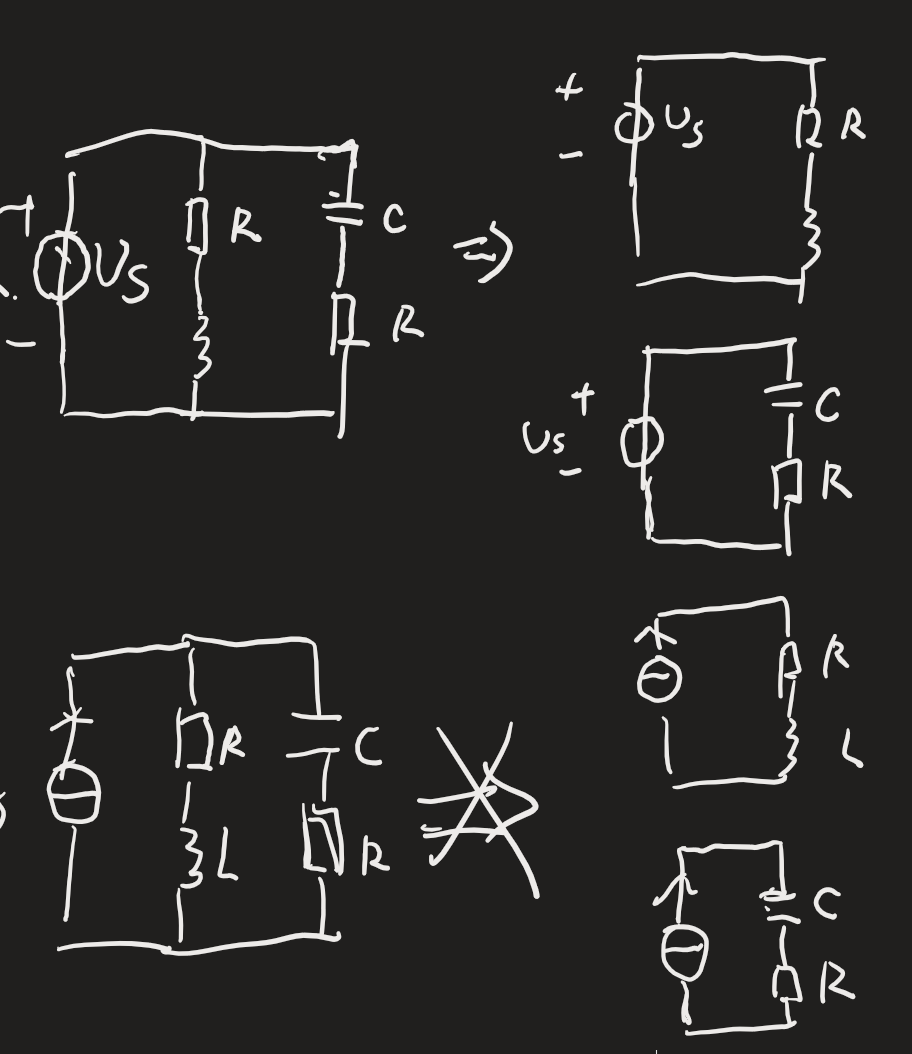二阶电路过渡过程的判定
在分析二阶电路时，先列出二阶常微分方程（可先从电路的状态方程入手）
ex：
$LC\frac{d^2u_c}{dt^2}+RC\frac{du_c}{dt}+u_c=0$
解常微分方程：
$LCp^2+RCp+1=0$
求得特征值p1、p2
1）若p1、p2为两个不相等的实根，则电路为非振荡放电过程（过阻尼），$R>2\sqrt{L/C}$
2）若p1、p2为两个相等的实根，为临界阻尼状态，$R=2\sqrt{L/C}$
3）若p1、p2为共轭复数根，电路为振荡放电过程（欠阻尼），$R<2\sqrt{L/C}$
冲激响应和阶跃响应
阶跃响应$ε(t)$
阶跃电源作用于电路时，通常先将激励的表达式写出来，然后根据叠加定理和齐次性求解即可。
冲激响应$δ(t)$
冲激电源激励的电路一般有两种分析方法：
1）分两个时间段考虑

t 在 0- 到 0+之间
以下图为例分析，且假设 uc(0-)=0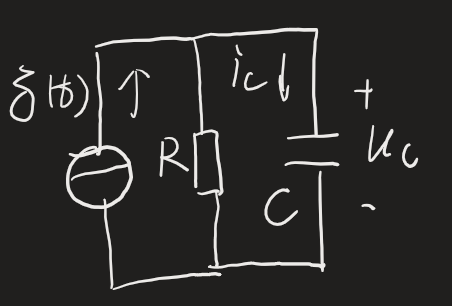电容充电，列方程
$C\frac{du_c}{dt}+\frac{u_c}{R}=δ(t)$
两边积分可得：
$\int_{0_-}^{0_+}C\frac{du_c}{dt}dt+\int_{0_-}^{0_+}\frac{u_c}{R}dt=1$
而uc为有限值，于是$\int_{0_-}^{0_+}\frac{u_c}{R}dt=0$
于是有：
$u_c(0_+)-u_c(0_-)=\frac{1}{C}$
又$u_c(0_-)=0$ 于是：
$u_c(0_+)=\frac{1}{C}≠u_c(0_-)$
t > 0+时为零输入响应
此时，电路只看右边回路
$u_c=\frac{1}{C}e^{-\frac{t}{RC}}$
$i_c=-\frac{u_c}{R}=-\frac{1}{RC}e^{-\frac{t}{RC}}$

又因为在冲激电流作用下 ic 跃变，于是有
$u_c=\frac{1}{C}e^{-\frac{t}{RC}}ε(t)$
$i_c=δ(t)-\frac{1}{RC}e^{-\frac{t}{RC}}ε(t)$
图像如下：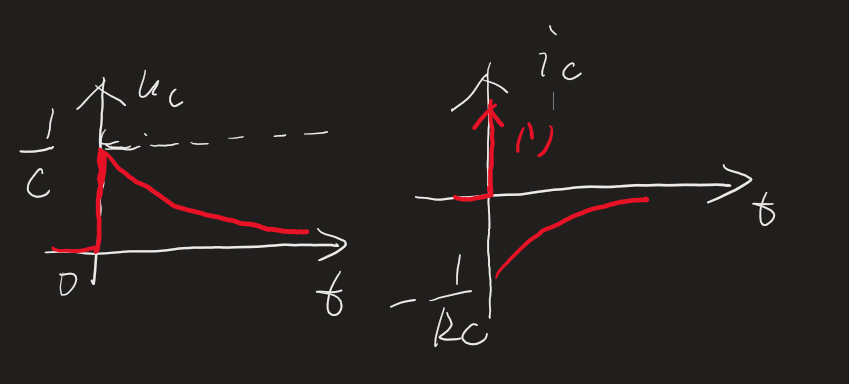同理可求如下RL电路的冲激激励响应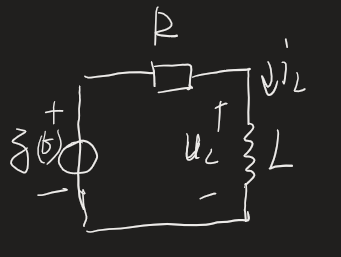2）先求阶跃响应

先令激励为ε(t)
三要素法分析电路（通常先求出uc和iL）
然后对第二步所求得量求导
根据$f(t)δ(t)=f(0)δ(t)$运算以及消去零项
整理即可得结果

参考：
邱关源电路第五版

欢迎指正，侵删


展开全文• 相对来说，乘积要比卷积的积分要温柔的多，然后我们在S域里面得到结论过后，再将其反映射回到时域，然后自然地在时域使用其所得的结论了。 以下仅举两个例子，就一阶惯性惯性滤波器和二阶滤波器的算法实现做简要介绍...
• 电路理论实验指导 戴维南 叠加定理 一阶电路 伏安特性测量等实验 1．研究ＲＣ一阶电路的零输入响应、零状态响应和全响应的规律和特点； 2．学习一阶电路时间常数的测量方法，...3．掌握微分电路和积分电路的基本概念。
• 一阶电路的零输入响应 一阶电路的零状态响应 一阶电路的全响应 二阶电路的零输入响应 二阶电路的零状态响应和全响应 一阶电路和二阶电路的阶跃响应 一阶电路和二阶电路的冲激响应 卷积积分 状态方程 动态电路时域...
• RC一阶电路的响应测试--实验报告 233333333333333333333333333333333333333333333333
• 最近有不少同学再问我们微信公众号里的移相电路，而且都希望工作在较高频率，我估计是不是因为马上电子设计竞赛了，有同学想用此电路结构做一个通信上的调相电路，有了调相电路，把调制信号积分一下就变成了调频电路...
• 一阶电路是简化后只含有一个电容或电感元件的电路，而在这些电路中，常常会需要分析开关断连前后的情况，这就需要对电路做瞬态分析
• 一阶的RC高低通电路和微积分电路有什么区别啊？就比如积分电路和低通电路，感觉在传递函数上是一样的啊，我用积分电路写个程序，时域上的波形按公式积分，怎么得不到想要的结果呢，可能是程序写错了，但是还是对这...matlab 经验分享
• 移相电路就是对输入信号(一般是正弦波)进行相位控制，而不改变其幅度，本推文以移相电路为例，展示模拟电路的反馈设计技巧与方法：一、全通滤波器实现移相以上是两种移相电路 的原理，其输出幅度保持不变，移动的...
• 由于非直流及非正弦信号激励下的一阶线性动态电路的全响应不能直接、简单地应用一阶电路三要素法来求 解,在此从一阶电路微分方程出发,通过构建一个恰当的微分,然后积分再求其解,得出任意信号激励下一阶电路全响应 的...
• 先来简述一阶系统(也可直接跳转至2部分)积分器+单位负反馈组成的一阶系统框图闭环传递函数框图的伯德图阶跃响应阶跃响应(时域响应)带宽处幅值下降3dB≈√2增益-带宽积一节运放的传递函数单位增益频率(unit-gain ...
• 运算电路 5. 应用拉普拉斯变换法分析线性电路 6. 网络函数的定义 7. 网络函数的极点和零点 8.极点、零点与冲激响 9.极点、零点与频率响应 01—拉普拉斯变换的定义拉普拉斯变换分为正变换和反变换，有多个动态元件...
• 通过分析动力电池端电压与欧姆极化、电化学极化、浓差极化、不平衡电势、迟滞特性等因素之间的关系，确定动力电池的内部状态变量，提出动态综合型等效电路模型(DS-ECM)，借助混合脉冲功率特性法(HPPC)进行动态辨识。...
• 【作文原因】：记得刚学习到电路原理正弦稳态分析这一板块的时候，当时我的内心是崩溃的。走马观花的在高处随手胡乱用头上加点的电流电压求解相关未知量并没有使我感到一丝成就。相反，我在运用“科技黑箱”的同时...
• 动态电路中无外施激励电源，仅由动态元件初始储能所产生的响应，称为动态电路的零输入响应。 RC电路的零输入响应 　开关S闭合前，电容C已充电，其电压uC=Uo。现把开关动作时刻取为计时起点(t=0)。开关闭合后，即...
• 自动化人 - 知乎​www.zhihu.com金鸡一唱天下白：第六讲 三相交流电路分析​zhuanlan.zhihu.com7.1 线性电路的过渡过程由理想线性元件构成的电路，如电感、电容等储能元件组成的电路，在某个初始条件下，储能元件...
• 一阶系统： 假设一个一般性的一阶系统传递函数模型为： 一阶系统中只有一个参数T（时间常数）也称为惯性环节，所以我们研究改变T会如何影响系统的输出c（t）。 解出时间响应 所以得到了输出与参数T的关系，那么我们...
• 一阶电路:描述电路的方程是一阶微分方程,一阶电路中一般只有一个动态元件 二阶电路:描述电路的方程是二阶微分方程,一般有二个动态元件 ; 应 用 K V L : R i + u c = u s 电 容 V C R : i = C d u c d t R C d u...
• 一阶系统的频率响应 一阶系统： 当 当 当 所以一阶系统的频率响应是一个低通滤波 总结： 无论是室内空调系统、流体系统还是含电容器的电路系统，容器就是一个缓冲器，其本质是抑制高速变化。缓冲也会带来延迟。 ...
• 解析微分电路和积分电路的区别 http://www.elecfans.com/dianzichangshi/20170515516185.html 简单理解RC 积分电路 CR微分电路 https://www.dgzj.com/dianzi/85307.html 如何区分积分电路和微分电路 ...
• 积分电路和微分电路的特点 1:积分电路可以使输入方波转换成三角波或者斜波 微分电路可以使输入方波转换成尖脉冲波 2:积分电路电阻串联在主电路中，电容在干路中 微分则相反 3：积分电路的时间常数t要大于或者...
• 　输入为正弦信号，假设为Vi= Vmsin(ωt + φ)，对于下面一阶积分电路而言，根据电压定律可得: 　其中，Vi1=（1+VR）Vi/2，R1=2R/（1+VR），Vi1和R1分别是等效输入电压和电阻。同理，Vi1=（1-VR）Vi/2，R1=2R/...
• 输出信号与输入信号的积分成正比的电路：积分电路 输出信号与输入信号的微分成正比的电路：微分电路 1)一阶RC低通滤波器 RC低通滤波器的电路及其幅频、相频特性如下图所示。 设滤波器的输入电压...网络
• 积分运算放大电路积分电路是使输出信号与输入信号的时间积分值成比例的电路，积分电路主要用于波形变换、放大电路失调电压的消除及反馈控制中的积分补偿等场合。积分电路是一种应用比较广泛的模拟信号运算电路。它是...
• ## RC微积分电路

万次阅读 2010-06-08 13:47:00
9 RC一阶电路（动态特性 频率响应）    一个电阻和一个电容串联起来的RC电路看起来是很简单的电路。实际上其中的现象已经相当复杂，这些现象涉及到的概念和分析方法，是电子电路中随处要用到的...网络 工具 c...